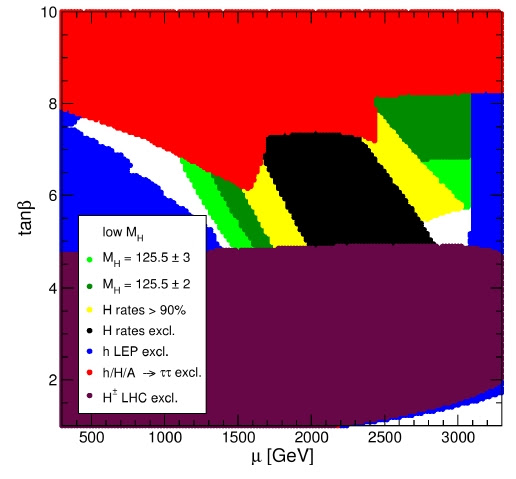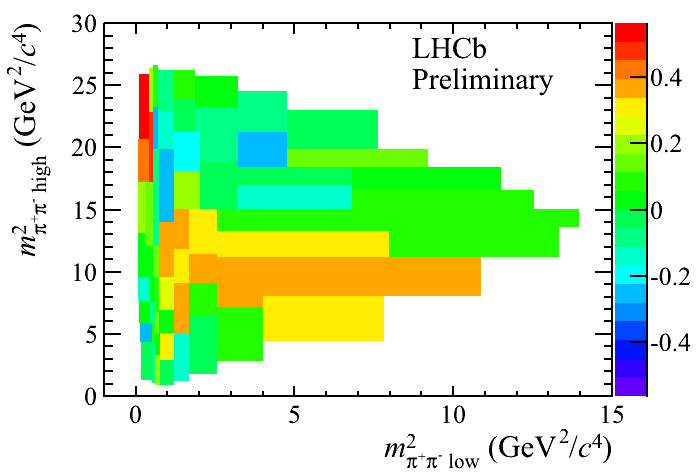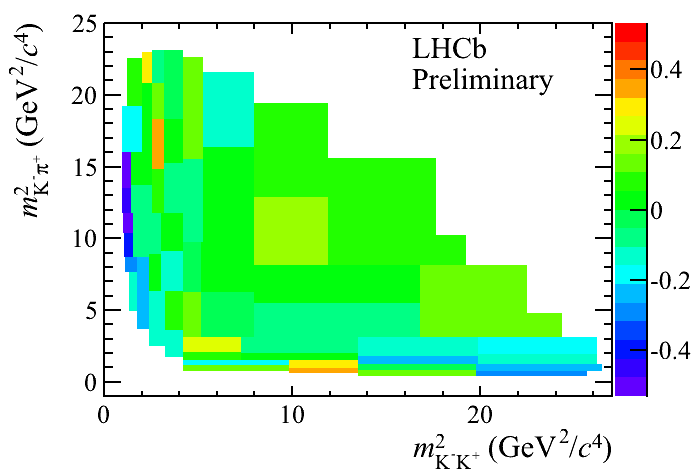## Monday, March 04, 2013 ...//### LHCb: $$7$$-$$\sigma$$ and $$9$$-$$\sigma$$ anomalies in CP-violation

First, let me start with Tommaso Dorigo. He reviewed a recent paper by Carena et al. that tried to find the best parameters of the MSSM Higgs sector that are compatible with the LHC experiments so far. Dorigo reprints this graphfor a particular "low $$M_H$$" scenario (subset of MSSM possibilities) where the green bands (especially the dark green band) are still allowed. He concludes by saying

All in all one gets the impression that the "window of opportunity" for the MSSM is closing down. But if you read the paper (written by MSSM enthusiasts) you might get a different idea!
What a dumb criticism of the paper!

The actual reason why you might get a different idea is that neither the authors of the Carena et al. paper nor you are imbeciles controlled by deluded impressions. The right interpretation is that aside from the binary uncertainty (the fact that the allowed regions survive at two places), the LHC is measuring the parameters $$\mu$$ in the low-$$M_H$$ scenario more accurately than before. The scenario doesn't have to be right but the data are encouraging for its future.

The ratio of the two Higgs vevs is about $$4\leq \tan\beta\leq 8$$ while $$\mu$$ either belongs to $$(1200\GeV,1600\GeV)$$ or $$(2500\GeV,3000\GeV)$$ or so. If Dorigo had at least some memory, he would know that just a year (or a year and a half) ago, the Higgs boson was almost exactly in the same situation. For example, in March 2012, the Tevatron confirmed that the Higgs could only exist in the 115-135 GeV interval. The LHC experiments were able to confine the "window of opportunity" to a similar interval several months earlier.

Were the windows of opportunity closing down? No. In March 2012, we actually already knew that the $$124-126\GeV$$ Higgs would be officially discovered within half a year and it turned out to be the case, indeed. It's not surprising that the wrong values are excluded before the right value is officially discovered. After all, we are used to excluding values at the $$2$$-$$\sigma$$ level but discovering things at the $$5$$-$$\sigma$$ level. These apparent "double standards" are followed for a good reason – because only extraordinary claims (about a new particle/effect) require extraordinary ($$5$$-$$\sigma$$) evidence; claims that no new effect with certain values of parameters exists isn't extraordinary so $$2$$-$$\sigma$$ evidence supporting this original "null hypothesis" is considered enough. So most typically, we first exclude almost all the wrong values of the parameters and then we discover the new thing and the right values of the parameters.

It was the case of the Higgs boson and only a foolish victim of circular reasoning could assume that it can't happen again with the supersymmetric particles. Dorigo established that the "windows are closing down" because he has assumed that the windows should close down from the beginning. But nothing like that is indicated by the actual data which show that the low $$M_H$$ scenario of the MSSM is alive and kicking and it has become highly predictive. In fact, the graph above shows how incorrect are the claims of the anti-supersymmetric crackpots who often say that all the parameters are being constantly sent somewhere to the infinity where the new effects are invisible. It ain't the case. The LHC experiments indicate that the right place is in the left middle or right middle of the graph.

But let me talk about someone who is less deranged than Tommaso Dorigo.

Adam Davis of the U.S. LHC blogs dehibernated himself and wrote about
A Puzzling Asymmetry.
In Fall 2012, the LHCb collaboration presented preliminary results at a CKM conference. They investigated three-body decays of B-mesons:$\eq{B^\pm &\to \pi^\pm \pi^+\pi^-\\ B^\pm &\to \pi^\pm K^+ K^- }$ For each possible momenta of the three final decay products, they measured the CP-asymmetry (proportional to the difference between the decays of one type and the CP-transformed process). And all these decay events were captured by the Dalitz plot, a useful visualization technique invented by R.H. Dalitz 60 years ago.

When a particle decays to two final products, the energy and (the magnitude of) the momentum of each decay product (measured in the rest frame of the initial particle) is determined by the energy-momentum conservation. Imagine a Higgs boson going to two photons: each photon inherits one-half of the Higgs latent energy. However, for three-body decays (such as the three-meson decays of the B-mesons above), there is some freedom how the energy and momentum may be distributed.

Let me do the counting of parameters. For two decay products, there are $$2\times 4$$ components in the final energy-momentum vectors, $$2$$ on-shell conditions, and $$4$$ components of the energy-momentum conservation law. We get $$2\times 4-2-4=2$$ parameters left – something that only determines the point on the sphere (direction of one particle; the other one moves oppositely; the rotational symmetry around the axis of their motion is unbroken) which is irrelevant due to the rotational symmetry, anyway. Both $$2$$ parameters are sacrificed for the rotational symmetry and no interesting freedom is left.

For three decay products, this counting is $$3\times 4 - 3 - 4=5$$ but $$3$$ parameters out of the $$5$$ describe a general $$SO(3)$$ rotation of the three bodies so they don't change the situation qualitatively. Only $$5-3=2$$ parameters label inequivalent energy-momenta of the decay products. They may be written as $$m^2_{\pi^+ K^-}$$ and $$m^2_{K^-K^+}$$ in the case of the decay involving the kaons. And these two parameters are axes in the Dalitz plot. The color in the Dalitz plot may denote the relative contribution of decay events with some particular "geometry of the momenta and distribution of the energies" to the asymmetry or something of the sort.

The resulting pictures look like this:The Dalitz plots tell us more than just "one overall number" quantifying the asymmetry. They inform us which final geometries of the energy-momentum vectors of the three decay products are dominant in the asymmetry.

I don't want to bore you with precise numbers but there are puzzling regions in which the asymmetry exceeds $$|A|\gt 0.6$$ and the statistical significance of these deviations is over $$7$$-$$\sigma$$ and $$9$$-$$\sigma$$, respectively! This is clearly way too large even after you punish these statistical significances by the look-elsewhere reduction.

The invariant masses of the pairs of mesons where this shocking thing occurs are either several $$\GeV$$s or around $$15\GeV$$. Bizarre. Next time when you hear that the LHCb excludes all new physics, don't forget that quite some breathtaking results have been swept under the rug if not fully censored when such a bold negative statement was made.

In the aggressive case, these asymmetries could be signs of some new – perhaps supersymmetric – particles. Perhaps the same particles that make up the dark matter and that could be announced this or next week. Perhaps sgluinos in some extended gauge sector. Adam Davis proposes a conservative competing theory involving "just a partial interference" of the quantum amplitudes for different processes. I am not sure I understand what it means even though I also believe that the deviation is pretty likely to be due to some incorrect calculation of the predictions, a forgotten subtlety.

It could be fun – and an example of a discovery that could force us to say, sometime in the future, that "we must have been blind and stupid if we were overlooking these complete and unquestionable $$7$$-$$\sigma$$ and $$9$$-$$\sigma$$ proofs".#### snail feedback (4) :reader Dilaton said...

Oh Lumo,

you are very brave to still having the stomach to keep clicking and reading Tommaso Dorigo ;-)

I have definitively and completely stopped clicking him some time around Easter last year, after a very scornful and sarcastic post had appeared on his blog below a title involving the words "Easter lamb" and "SUSY", which clearly revealed Tommaso Dorigo's true colors and similarity (or indistinguishability) to the Trollking and attracted the same scum in the comments etc ...

So I am not in the slightes surprised by his sourballish attitudes concerning the the results presented in the new SUSY paper. But every dimwit who is not an abominable sourball, notes the similarity of the narrowing down of the allowed parameter space to what happend before the discovery of the higgs.

It would be great fun, if these CP violation signals were due to some new cool physics. But I rather think too that it is more likely due to some more conservative issues ...

Maybe I should reconsider some stuff I have read, but I am not sure if I have encountered "sgluinos" before :-/ ... ? I mean the gluino is the superpartner of the gluon, but what exactly are sgluinos?

Maybe I am just confused because it is too late ...reader anna v said...

"It was the case of the Higgs boson and only a foolish victim of circular
reasoning could assume that it can't happen again with the
supersymmetric particles".

In the past we were not in the habit of making exclusion diagrams at all. Had we made exclusion diagrams on the road to all the discoveries leading to the standard model we would have been also excluding huge tracts of phase space. Some people should read what they are going to put up in a blog before they do so.

reader Luboš Motl said...

Exactly, Anna, it's so unremarkable to exclude possible theories - even big chunks of theories - simply because a vast majority of random things with random precise values of parameters we may invent don't exist!

reader Luboš Motl said...

Right, Dilaton! I still don't understand where this sourballism is coming from. SUSY, and some other ideas, is a wonderful, beautiful, and viable skeleton so closely resembling - but with some extra modern flavor - so many other cool things in the history of physics that turned out to be right.

What leads the sourballs to "wish" that those things are shown wrong? Of course that things may be wrong before they're fully proved. But there's a very strong case here and if true, this is a huge revolution in physics.

(function(i,s,o,g,r,a,m){i['GoogleAnalyticsObject']=r;i[r]=i[r]||function(){ (i[r].q=i[r].q||[]).push(arguments)},i[r].l=1*new Date();a=s.createElement(o), m=s.getElementsByTagName(o);a.async=1;a.src=g;m.parentNode.insertBefore(a,m) })(window,document,'script','//www.google-analytics.com/analytics.js','ga'); ga('create', 'UA-1828728-1', 'auto'); ga('send', 'pageview');